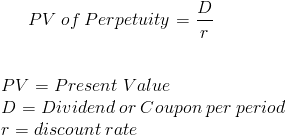# Present Value of Deferred Perpetuities

14th February 2020

A perpetuity is a type of annuity that receives an infinite amount of periodic payments. An annuity is a financial instrument that pays consistent periodic payments. As with any annuity, the perpetuity value formula sums the present value of future cash flows.

Common examples of when the perpetuity value formula is used is in consols issued in the UK and preferred stocks. Preferred stocks in most circumstances receive their dividends prior to any dividends paid to common stocks and the dividends tend to be fixed, and in turn, their value can be calculated using the perpetuity formula.

The value of a perpetuity can change over time even though the payment remains the same. This occurs as the discount rate used may change. If the discount rate used lowers, the denominator of the formula lowers, and the value will increase.

It should be noted that the formula shown supposes that the cash flows per period never change.